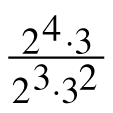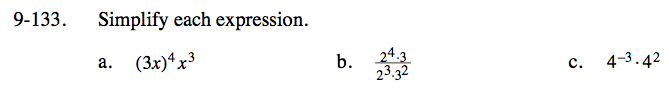### Home > CC3 > Chapter 9 > Lesson 9.2.5 > Problem9-133

9-133.
1. Simplify each expression. Homework Help ✎

1. (3x)4x3

2.3. 4−3 · 42Since the 3x is in parentheses, the 4 in the exponent applies to both the 3 and the x.

81x4x3

81x(4 + 3)

81x7

(2(4 − 3)) · (3(1 − 2))

21(3−1)

$\frac{2}{3}$

See (a) and (b).